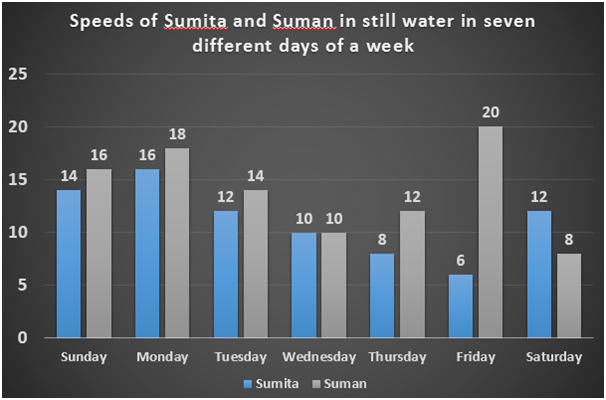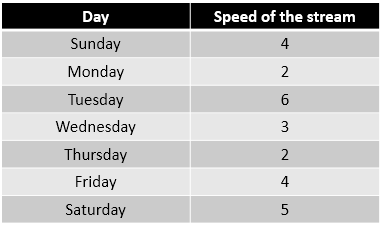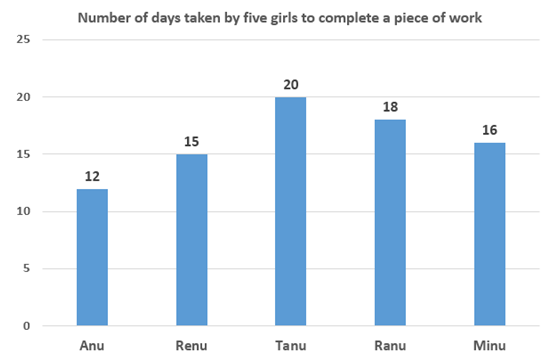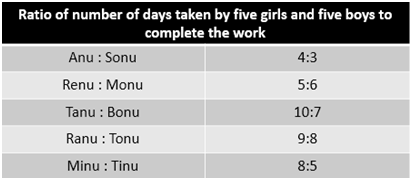# Quantitative Aptitude Questions (Data Interpretation) for SBI PO Mains 2018 Day-143

Dear Readers, SBI is conducting Online Examination for the recruitment of probationary officer. To enrich your preparation here we have providing new series of Data Interpretation – Quantitative Aptitude Questions. Candidates those who are appearing in SBI PO Mains Exams can practice these Quantitative Aptitude average questions daily and make your preparation effective.

[WpProQuiz 2918]

Click “Start Quiz” to attend these Questions and view Solutions

Directions (1 – 5): Study the following information carefully and answer the questions given below:

In the following bar graph and table, all the data are given in Km/h.1) Time taken by Sumita to travel 210 km upstream on Monday is what percent of the time taken by Suman to travel the same distance downstream on the same day?

a) 210%

b) 130%

c) 143%

d) 164%

e) None of these

2) Find the difference between upstream distance travelled by Sumita in 9 hours on Sunday, Monday and Tuesday together and downstream distance travelled by Suman in 8 hours on Sunday, Monday and Tuesday together.

a) 210 km

b) 170 km

c) 240 km

d) 130 km

e) None of these

3) Find the respective ratio of sum of downstream speeds of Sumita on Wednesday and Thursday and sum of upstream speeds of Suman on Friday and Saturday.

a) 23:19

b) 25:17

c) 19:23

d) 17:25

e) None of these

4) Upstream distance travelled by Sumita in 5 hours in all the days together is what percent of the upstream distance travelled by Suman in 3 hours in all the days together?

a) 103.13%

b) 105.23%

c) 120.37%

d) 111.37%

e) None of these

5) Find the sum of the time taken by Sumita to travel 160 km downstream in all the days together.

a) 78.14 hours

b) 82.56 hours

c) 80.35 hours

d) 66.24 hours

e) None of these

Directions (6 – 10): Study the following information carefully and answer the questions given below:6) Find the number of days taken by Anu, Ranu, Monu and Bonu together to complete the work.

a) 6(49/67) days

b) 4(52/67) days

c) 5(55/67) days

d) 3(51/67) days

e) None of these

7) Efficiency of Anu and Renu together is what percent of efficiency of Tanu and Ranu together?

a) 142.105%

b) 110.345%

c) 132.102%

d) 98.134%

e) None of these

8) Find the respective ratio of number of days taken by Sonu and Monu together to complete the work and number of days taken by Bonu and Tonu together to complete the work.

a) 47:34

b) 45:56

c) 56:45

d) 34:47

e) None of these

9) Renu and Minu started the work together and left after 5 days. In how many days Tonu and Tinu together can complete the remaining work?

a) 81/17 days

b) 85/17 days

c) 81/39 days

d) 85/39 days

e) None of these

10) Find the number of days taken by Sonu, Monu, Bonu, Tonu and Tinu together to complete the work.

a) 3(1020/2017) days

b) 3(1002/2019) days

c) 2(1002/2017) days

d) 2(1002/2019) days

e) None of these

Direction (1-5)

Upstream speed of Sumita on Monday = 16 – 2 = 14 km/h

Time taken by Sumita to travel 210 km upstream on Monday = 210/14 = 15 hours

Downstream speed of Suman on Monday = 18 + 2 = 20 km/h

Time taken by Suman to travel 210 km downstream on Monday = 210/20 = 10.5 hours

Required percentage = 15/10.5 x 100 = 142.8 %= 143%

Upstream speed of Sumita on:

Sunday = (14 – 4) = 10 km/h

Monday = (16 – 2) = 14 km/h

Tuesday = (12 – 6) = 6 km/h

Downstream speed of Suman on:

Sunday = (16 + 4) = 20 km/h

Monday = (18 + 2) = 20 km/h

Tuesday = (14 + 6) = 20 km/h

Upstream distance travelled by Sumita in 9 hours on Sunday, Monday and Tuesday together

= 10 x 9 + 14 x 9 + 6 x 9

= 90 + 126 + 54

= 270 km

Downstream distance travelled by Suman in 8 hours on Sunday, Monday and Tuesday together

= 20 x 8 + 20 x 8 + 20 x 8

= 160 + 160 + 160

= 480 km

Required difference = 480 – 270 = 210 km

Downstream speed of Sunita on:

Wednesday = 10 + 3 = 13 km/h

Thursday = 8 + 2 = 10 km/h

Sum of downstream speeds of Sumita on Wednesday and Thursday = 13 + 10 = 23 km/h

Upstream speed of Suman on:

Friday = 20 – 4 = 16 km/h

Saturday = 8 – 5 = 3 km/h

Sum of upstream speeds of Suman on Friday and Saturday = 16 + 3 = 19 km/h

Required ratio = 23:19

Upstream speed of Sumita on:

Sunday = (14 – 4) = 10 km/h

Monday = (16 – 2) = 14 km/h

Tuesday = (12 – 6) = 6 km/h

Wednesday = (10 – 3) = 7 km/h

Thursday = (8 – 2) = 6 km/h

Friday = (6 – 4) = 2 km/h

Saturday = (12 – 5) = 7 km/h

Upstream distance travelled by Sunita in 5 hours in all the days together

= 10 x 5 + 14 x 5 + 6 x 5 + 7 x 5 + 6 x 5 + 2 x 5 + 7 x 5

= 50 + 70 + 30 + 35 + 30 + 10 + 35 = 260 km

Upstream speed of Suman on:

Sunday = (16 – 4) = 12 km/h

Monday = (18 – 2) = 16 km/h

Tuesday = (14 – 6) = 8 km/h

Wednesday = (10 – 3) = 7 km/h

Thursday = (12 – 2) = 10 km/h

Friday = (20 – 4) = 16 km/h

Saturday = (8 – 5) = 3 km/h

Upstream distance travelled by Suman in 6 hours in all the days together

= 12 x 3 + 16 x 3 + 8 x 3 + 7 x 3 + 10 x 3 + 16 x 3 + 3 x 3

= 36 + 48 + 24 + 21 + 30 + 48 + 9 = 216 km

Required percentage = 260/216 x 100 = 120.37%

Downstream speed of Sumita on:

Sunday = (14 + 4) = 18 km/h

Monday = (16 + 2) = 18 km/h

Tuesday = (12 + 6) = 18 km/h

Wednesday = (10 + 3) = 13 km/h

Thursday = (8 + 2) = 10 km/h

Friday = (6 + 4) = 10 km/h

Saturday = (12 + 5) = 17 km/h

Required sum of the times = 160/18 + 160/18 + 160/18 + 160/13 + 160/10 + 160/10 + 160/17

= 8.88 + 8.88 + 8.88 + 12.30 + 16 + 16 + 9.41

= 80.35 hours

Direction (6-10)

Number of days taken by Monu to complete the work = 6/5 x 15 = 18 hours

Number of days taken by Bonu to complete the work = 7/10 x 20 = 14 hours

Let the number of days taken by Anu, Ranu, Monu and Bonu together to complete the work = x

x(1/12 + 1/18 + 1/18 + 1/14) = 1

=>x(21 + 14 + 14 + 18)/252 = 1

=> x = 252/67

=> x = 3(51/67) days

Number of days taken by Anu and Renu together to complete the work = (12 x 15)/(12 + 15)

= 180/27

= 20/3

Number of days taken by Tanu and Ranu together to complete the work = (20 x 18)/(20 + 18)

= 360/38

= 180/19

Efficiency ratio:

(Anu + Renu): (Tanu + Ranu) = 3/20: 19/180 = 27:19

Required percentage = 27/19 x 100 = 142.105%

Number of days taken by Sonu to complete the work = ¾ x 12 = 9 days

Number of days taken by Monu to complete the work = 6/5 x 15 = 18 days

Number of days taken by Bonu to complete the work = 7/10 x 20 = 14 days

Number of days taken by Tonu to complete the work = 8/9 x 18 = 16 days

Number of days taken by Sonu and Monu together to complete the work = (9 x 18)/(9 + 18)

= (9 x 18)/27

= 6 days

Number of days taken by Bonu and Tonu together to complete the work = (14 x 16)/(14 + 16)

= (14 x 16)/30

= 112/15 days

Required ratio = 6 : 112/15

= 90:112

= 45:56

Number of days taken by Tonu to complete the work = 8/9 x 18 = 16 days

Number of days taken by Tinu to complete the work = 5/8 x 16 = 10 days

Let the number of days taken by Tonu and Tinu together to complete the work = x

5/15 + 5/16 + x(1/16 + 1/10) = 1

=>x(5 + 8)/80 = 1 – 1/3 – 5/16

=> 13x/80 = (48 – 16 – 15)/48

=> 13x/80 = 17/48

=> x = 17/48 x 80/13

=> x = 85/39 days

Number of days taken by Sonu to complete the work = ¾ x 12 = 9 days

Number of days taken by Monu to complete the work = 6/5 x 15 = 18 days

Number of days taken by Bonu to complete the work = 7/10 x 20 = 14 days

Number of days taken by Tonu to complete the work = 8/9 x 18 = 16 days

Number of days taken by Tinu to complete the work = 5/8 x 16 = 10 days

Let the required number of days = x

x(1/9 + 1/18 + 1/14 + 1/16 + 1/10) = 1

=>x(560 + 280 + 360 + 315 + 504)/5040 = 1

=> x = 5040/2019

=> x = 2(1002/2019) days

Daily Practice Test Schedule | Good Luck

 Topic Daily Publishing Time Daily News Papers & Editorials 8.00 AM Current Affairs Quiz 9.00 AM Quantitative Aptitude “20-20” 11.00 AM Vocabulary (Based on The Hindu) 12.00 PM General Awareness “20-20” 1.00 PM English Language “20-20” 2.00 PM Reasoning Puzzles & Seating 4.00 PM Daily Current Affairs Updates 5.00 PM Data Interpretation / Application Sums (Topic Wise) 6.00 PM Reasoning Ability “20-20” 7.00 PM English Language (New Pattern Questions) 8.00 PM